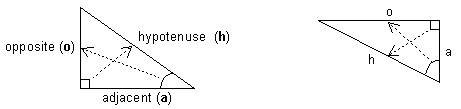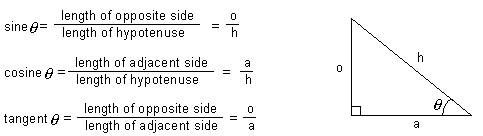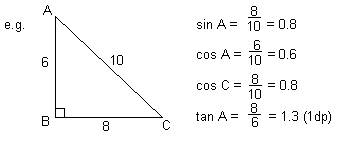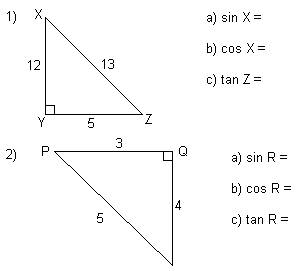# Trigometric Ratios Math Review and Tutorial

math tutorials > trig ratios math review

## Introduction to Trig Ratios

We can use Pythagoras' Theorem to calculate the length of an unknown side in a right-angled triangle when we are given information about the lengths of 2 other sides.
However, if we are given information about an angle (other than the 90 degrees) and one side and need to calculate the length of another side then we use the trig ratios.

## Labelling the sides of a right-angled triangle.

We already know the side opposite the right-angle is called the hypotenuse.  We label the other 2 sides according to their position relative to the known angle.## The trigonometric ratios.

The 3 trigonometric ratios are called sine (sin), cosine (cos) and tangent (tan).  These ratios apply to right-angled triangles.## Trigonometry problems for you to try.Solutions : 1) a) 0.4 (1dp)     b)  0.9 (1dp)     c)  2.4 (1dp)
2) a)  0.6     b)  0.8     c)  0.75